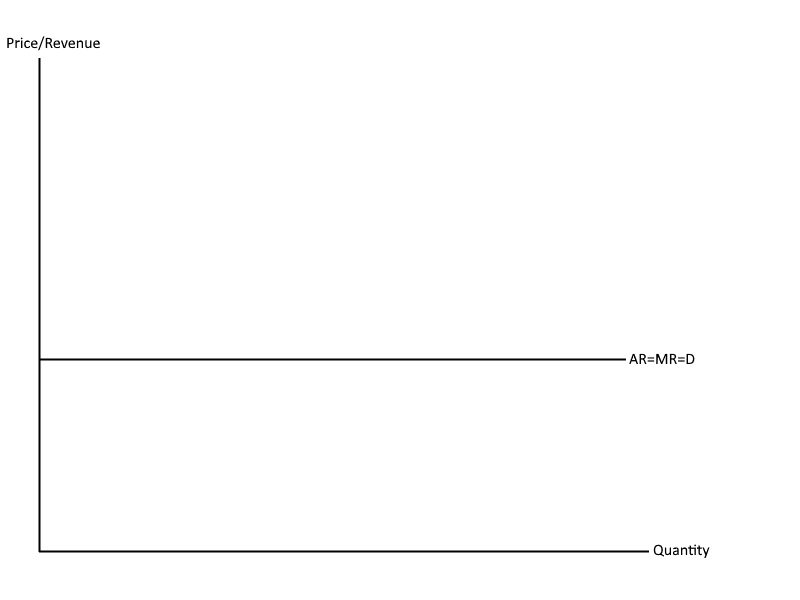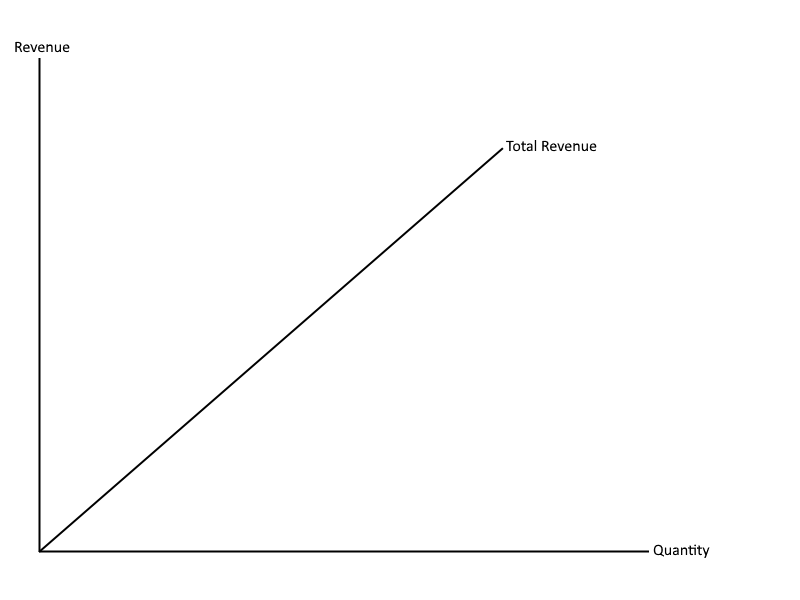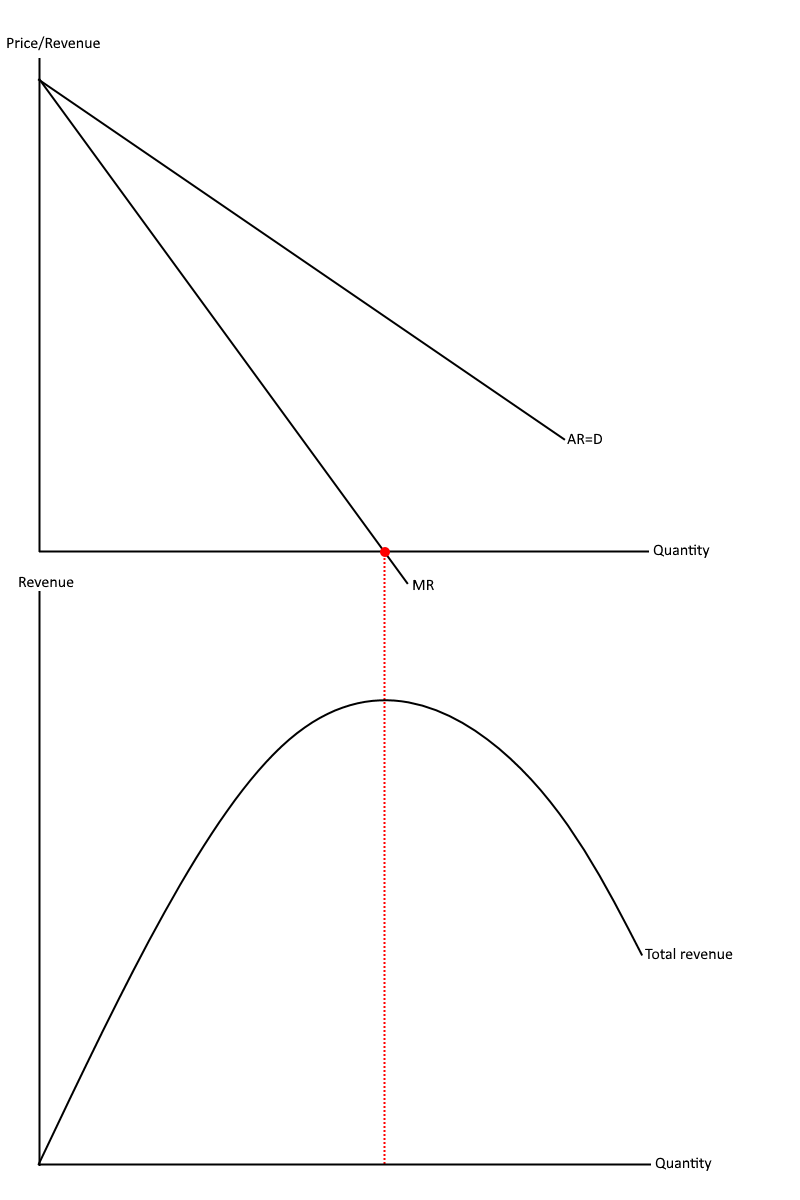# Revenue

## Revenue formulae

Total revenue (Price x quantity)

Average revenue (Total revenue/quantity)

Marginal revenue (Change in revenue/change in quantity)

## Revenue curves

Perfect competitionIn perfectly competitive markets there are a number of characteristics that result in firms in the market becoming price takers. This means that they have no influence over the price level they charge. Some of the characteristics include homogenous goods, many buyers and sellers and perfect information. If one firm were to charge a higher price than the other, the consumers in the market would know this. As a result of this, the firm charging the higher price would lose all their customers as they would now be buying the good/service from the firm selling it for a cheaper price. This explains the horizontal average revenue and marginal revenue line. The following example further explains this.

 Price Quantity Total Revenue Average Revenue Marginal Revenue 2 1 2 2 2 2 2 4 2 2 2 3 6 2 2

All, the prices within the market are the same, in this example that price is 2. The total revenue is equal to the price multiplied by the quantity and therefore the total revenue will consist of 2, 4 and 6. For each extra good/service produced, total revenue increases by 2. Therefore, the marginal revenue is 2. In addition to this, the average revenue (total revenue/quantity) for each unit is the same. As the average revenue and the marginal revenue are the same price and stay at that same price, both of which are portrayed by a horizontal straight line at the price of 2.As shown from the table, the marginal revenue is always 2. This means that the total revenue line on the diagram increases at the same rate and therefore has the same gradient.

Imperfect competitionIn imperfectly competitive market, firms are price makers. This is due to features such as different goods/services produced, barriers to entry and exit etc. As a result of this, the law of supply and demand are able to operate which is why, as the price level decreases, quantity increases.

 Price Quantity Total Revenue Average Revenue Marginal Revenue 10 1 10 10 10 9 2 18 9 8 8 3 24 8 6 7 4 28 7 4 6 5 30 6 2 5 6 30 5 0 4 7 28 4 -2 3 8 24 3 -4

As shown from the example above, both average revenue and marginal revenue decrease as the quantity increases. This explains why both revenue line are downwards slopping. However, marginal revenue is also decreasing twice as fast as average revenue, therefore this is shown on the diagram above by a marginal revenue line that is twice as steep as the average revenue line.

The table can also be used to explain the total revenue curve. At the price of 5 revenue maximization takes place as marginal revenue is equal to 0. This is shown by the red dot on the average and marginal revenue diagram. As this is the revenue maximization point, the dotted red line shows that this is also the point that the total revenue curve is at its highest. The table shows us that after this point marginal revenue starts to decrease, this is why the total revenue line starts to decrease after this point.

You will also notice that the total revenue line is increasing at a slower rate as it gets closer to the revenue maximization point. This is also shown on the table as marginal revenue gets less and less as quantity increases. After the revenue maximization point, marginal revenue starts to fall at a faster and faster rate as quantity increases. These two factors explain the parabola like curve of total revenue.

PED and revenue

When demand is price elastic a change in price will cause a more than proportionate change in quantity demanded. On the other hand, when demand is price inelastic a change in price will cause a less then proportionate change in quantity demanded. Knowing this we can make certain conclusions when it comes to the link between PED and revenue.When demand is price inelastic firms are better off increasing their prices. This can be seen on the diagram above. The increase in price (P1 to P2) causes a less then proportionate change in quantity demanded (Q1 to Q2). As a result of this, total revenue increases from 0-P1-A-Q1 to 0-P2-B-Q2. If the firm decided to decrease their prices instead, then total revenue would decrease. This is why it is important for firms to know whether they sell goods/services that are price inelastic or price elastic, as they can change their price to produce the optimal amount of total revenue.When demand is price elastic, a decrease in price (PQ to P2) will cause a more then proportionate change in quantity demanded (Q1 to Q2). As a result of this the total amount of revenue increases (0-P1-A-Q1 to 0-P2-B-Q2). If the firm were to increase the price of a price elastic good/service, then total revenue would fall.